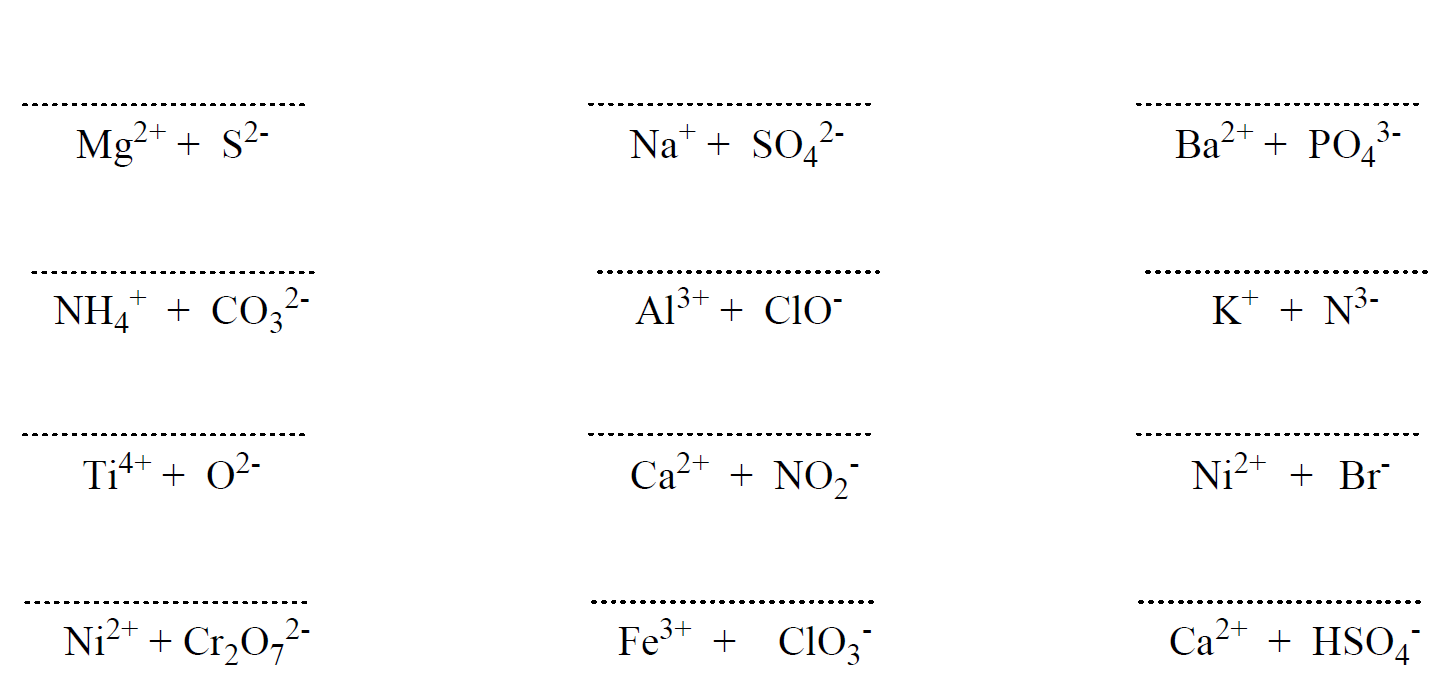## General Chemistry

The ionic bond is normally formed between metals and nonmetals and the reason for this is their different electron affinity. Remember, metals have low ionization energies and therefore, tend to form cations, while nonmetals have high electron affinity and tend to form anions. So, we can look at it his way; when mixing a metal with a nonmetal, the metal gives an electron(s) to the nonmetal which yields a cation and an anion: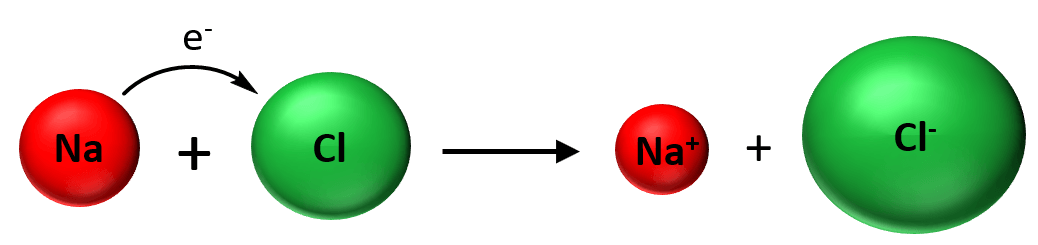Now, as soon as the ions are formed to stick together as a result of electrostatic attractive forces and is what we classify as an ionic bond: An ionic bond is an electrostatic force that holds ions together in an ionic compound.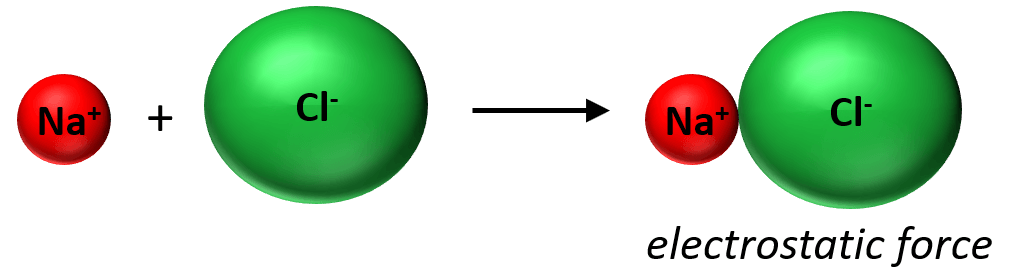What is important here is that we are not talking about the interaction of one Na+ and Cl+ ion together. Unlike covalent bonding, ionic bonds are not directional and specific between given atoms, but rather they form a lattice as an interaction among the entire cluster of the ions: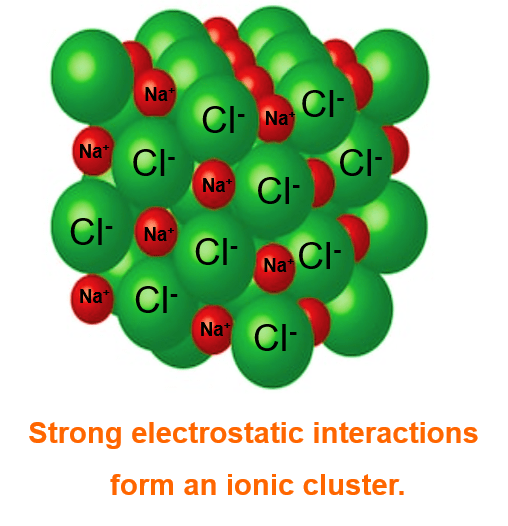In other words, there is not really such thing as one bond between two ions/atoms like in covalent compounds and that’s why they need a lot of thermal energy to break these interactions and therefore, have high melting points.

# Electron Configuration and Ionic Bonds

Let’s see how the formation of ionic bonds can be visualized by looking at the changes in electron configurations. First, we write the electron configuration of sodium and chlorine:

Na – 1s² 2s² 2p 3s¹

Cl – 1s² 2s² 2p 3s² 3p

Now, chlorine, having a higher electron affinity, takes one electron from the sodium and this electron is the one in the highest energy level. In this case, it is the one electron in the 3s orbital.

1s² 2s² 2p 3s¹  → 1s² 2s² 2p + e

Na → Na+

The electron is transferred to the 3p orbital of chlorine where there was only one electron and therefore, the 3p5configuration changes to 3p6:

1s² 2s² 2p 3s² 3p+ e–  → 1s² 2s² 2p 3s² 3p6

Cl → Cl

Using Lewis dot symbols, we show this transformation as:Notice that both the Na+ and Cl- ions have an electron configuration of a noble gas:

Na+ – 1s² 2s² 2p = [Ne]

Cl– 1s² 2s² 2p 3s² 3p6 = [Ar]

Now, remember that noble gases are also called inert gases as they are very stale and unreactive. This has to do with the stability of completely filled electron shells and it explains why, for example, Na and other alkali metals in group 1 do not become 2+ or 3+ charged. Likewise, we can explain why oxygen becomes 2 and Al becomes 3+ when forming ionic compounds.

Let’s see how the electron configurations change during the formation of Al2O3 using Lewis dot symbols.Al is in group 3 and therefore, it tends to lose 3 electrons adapting the electron configuration of a noble gas Ne. Oxygen, on the other hand, can acquire 2 electrons in order to get the electron configuration of Ne. Therefore, the process involves a transfer of 6 electrons where two Al atoms are losing 3 electrons each, and 3 oxygen atoms are gaining 3 electrons each.

# A Shortcut to Predicting the Formula of Ionic Compounds

To predict the formula of an ionic compound, remember first that metals tend to lose, and nonmetals tend to gain as many electrons as needed to adapt an electron configuration of a noble gas closest to them in the periodic table. Metals gain change based on their group number. Metals in group 1 become 1+, those in group 2 become 2+, and the ones in group 3 become 3+.

For example, what would be the formula of the compound when Mg reacts with N2?

Magnesium is in group 2, therefore, it will become 2+ changed. Nitrogen is in group 5, and therefore, it will need 3 electrons to adapt the electron configuration of the noble gas that comes next in the periodic table which is Ne. This makes is 3- charged, so we have Mg2+ and N3- ions. Now, put the ions next to each other and cross down the charges to get the correct subscript for each element: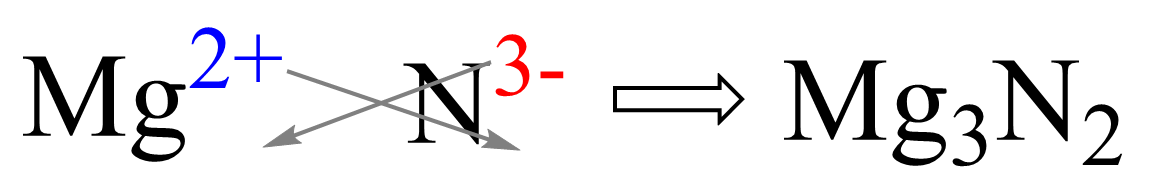Check Also

Check this 90-question, Multiple-Choice Quiz on Chemical Bonding:

#### Practice

1.

Write the formulas of the compounds formed from the following ions: